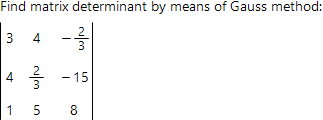# Matrix determinant online calculatorMatrix determinant is the number, which by definitive rule can be set in one-to-one correspondence to any square matrix.

There are many ways to find matrix determinant. This online calculator supports Gauss method and the method of the determinant decomposition by choosen row or column.

To find determinant by Gauss method initial matrix by elementary transformations simplified to row reduced form. Elementary transformations don't change the value of the determinant. At the final stage of Gauss method, determinant is calculated as the product of the elements of main diagonal of row reduced matrix.Determinant of matrix A calculated by the formula:

| A | = a11a22 ∙ ... ∙ an−1 n−1ann

To find matrix determinant by means of row or column decomposition, one should initially choose the row or the column for decomposition. The most convenient way is to choose the row (or column) with maximum of its elements being equal to zero. If there are no such rows or columns, then any row or column can be choosen.Below, we present calculation of matrix B, determinant by means of decomposition by elements of the first row:Such a decomposition consists of the linear combination of determinants of lower orders compared by initial one. Every of such determinants can be again calculated by means of decomposition. Therefore, the method involved, represents recursive process.

Matrix determinant calculatorA =